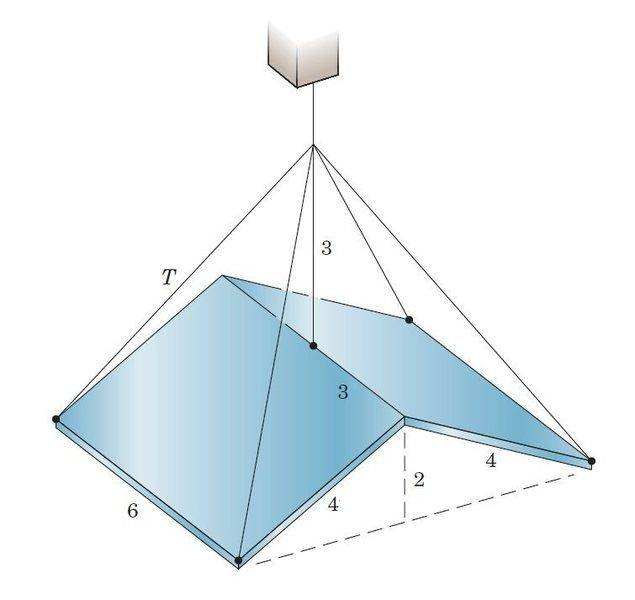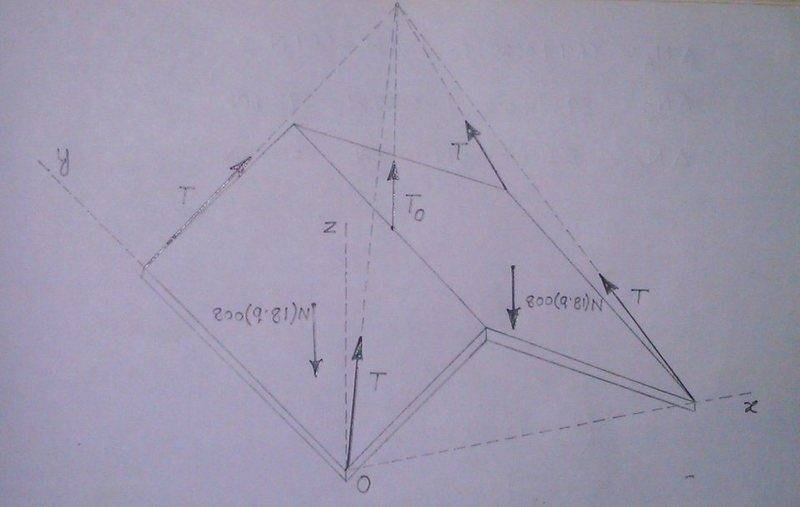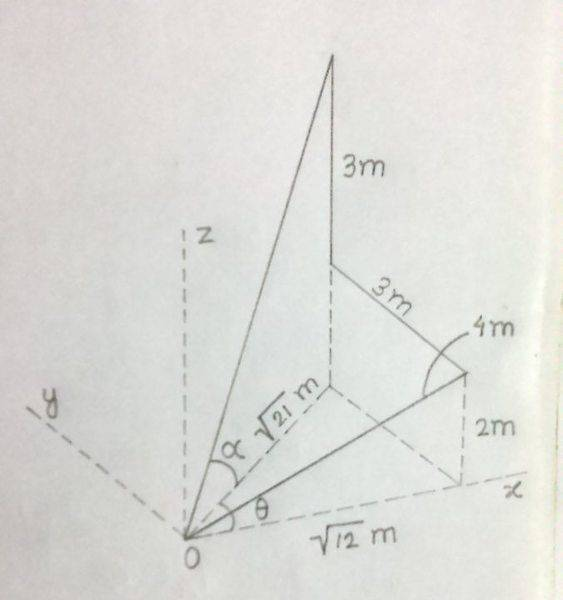# Statics: Problem about Equilibrium in 3-dimensions

## Homework Statement

The two uniform rectangular plates each weighing 800 kg are freely hinged about their common edge and suspended by the central cable and four symmetrical corner cables. Calculate the tension T in each of the corner cables and the tension T0 in the center cable.All dimensions in the figure are in meters.

2. Homework Equations

The scalar force equilibrium equations in three mutually perpendicular directions x-,y- and z-,i.e,

ΣFx=0 ΣFy=0 and ΣFz=0

The scalar moment equilibrium equations about three mutually perpendicular axes through a point,i.e,

ΣMx=0 ΣMy=0 and ΣMz=0[/B]

## The Attempt at a Solution

The Free Body Diagram of the plate assembly is drawn below along with the choice of the coordinate axes.The tensions in the corner cables 'T' can be resolved into its horizontal and vertical components as Txy and Tz respectively.The horizontal component Txy can then be resolved into components along x- and y- directions as Tx and Ty respectively in the x-y plane.The angles which orient the line of action of a corner cable tension T can be determined as illustrated in the figure below.The force equilibrium equations in the x- and y- directions are already satisfied since the identical x- and y- components of all the corner cable tensions cancel each other.

The force equilibrium equation in the z-direction yields,

ΣFz=0

⇒4Tsinα+T0-2.800(9.81)=0

⇒4T.##\frac {5}{\sqrt {46}}##+T0=15696

⇒2.95T+T0=15696

The moment equilibrium equation about the x-axis through O gives,

ΣMx=0

⇒2Tsinα(6)+T0(3)-2.800(9.81)(3)=0

⇒12T.##\frac {5}{\sqrt {46}}##+3T0=47088

⇒8.85T+3T0=47088

This is the same equation as obtained from ΣFz=0.

The moment equilibrium equation about the y-axis through O gives,

ΣMy=0

⇒ -2Tsinα(2##\sqrt {12}##)+800(9.81)(##\sqrt {12}##+##\frac {\sqrt {12}} {2}##)-T0(##\sqrt {12}##)+800(9.81)(##\frac {\sqrt {12}} {2}##)=0

-2T.##\frac {5} {\sqrt {46}}##.(2##\sqrt {12}##)+800(9.81)(##\sqrt {12}##+##\frac {\sqrt {12}} {2}##+##\frac {\sqrt {12}} {2}##) -T0(##\sqrt {12}##)=0

⇒ 10.22T+##\sqrt {12}##T0=54372.5
which is again the same equation as obtained before.

The moment equilibrium equation about the z-axis through O is already satisfied.
So as it is evident the equations of equilibrium are resulting into a single equation in T and T0.We need at least two dissimilar equations in T and T0 to solve for T and T0.

Where is the problem?
[/B]

haruspex
Homework Helper
Gold Member
2020 Award
Where is the problem?
All of your equations would still be true if the pair plates were to form a single rigid body. You need one which depends on their being hinged.

•Soumalya
All of your equations would still be true if the pair plates were to form a single rigid body. You need one which depends on their being hinged.
I had a thought about this approach earlier when a couple of things pushed me into doubts.Firstly,if we treat the two plates hinged together separately as two different bodies should we consider the effect of the centre cable on both the plates?

Secondly,if instead of being hinged together about their common edge the plates were welded together to form the assembly as shown(so that they could be treated as a single rigid body) would it be possible to determine the values of the tensions in that case using the conditions for static equilibrium?